﻿ Comp310 Lec11

# Lec11: Factories, cont.  & Transforming How We Paint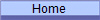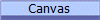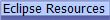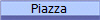First, let's finish our discussion on using factories to abstract the construction process of our updating strategies...

### Now, for some more fun.

Is updating the state of the ball the only place where strategies might be useful?    Take a look at the Fishworld Demo (We will do command dispatching shortly.  Command dispatching is separate from the painting features in Fishworld.)

## Affine Transforms

How does Fishworld create fish that are not only the correct size, but are always swimming forward?   How does it create different sizes and orientations of the same image?

Remember that a fish (ball) knows where it is and which way it is going because it has a position and a velocity.   It has a radius too, so it knows its size as well.   Thus any given ball can take a common representation of an image, e.g. a fish, scale it as per its radius, rotate it as per its velocity and translate it as per its position and end up with an image that is always oriented correctly with respect to its movement.    The mathematical process of scaling, rotating and translating is called an "affine transform".

An affine transform is the mathematical process of scaling (making larger or smaller), rotating (pivoting around the origin) and translating (moving a specified distance and direction) of a point in space.  An affine transform is a single operation that does all three transformations.  Remember that a point (coordinate in space, e.g. java.awt.Point) is really a vector from the origin, so a affine transformation technically transforms vectors, not geometrical points.

Affine transforms may seem mathematically daunting, but they are actually quite straightforward.   Plus, Java provides an AffineTransform class that encapsulates the mathematics and makes dealing with affine transformation very easy.   Translation:  we never have to fuss with the math, we just use the results.   Gotta love that!

Example of the operations involved in a single Affine transformation, showing the individual steps of rotating, scaling and translating:

Original vector: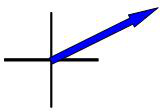then Rotated vector: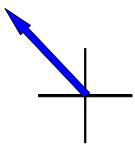then Scaled vector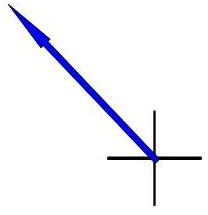and then Translated vector: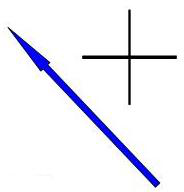Note that the order of operation is important:

Original vector: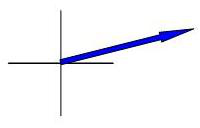then Rotated vector: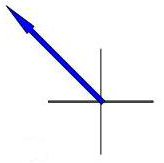then Translated vector: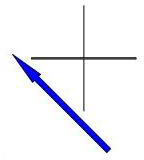as compared to

Original vector: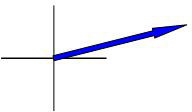then Translated vector: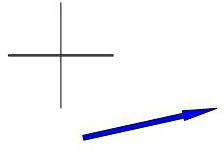then Rotated vector: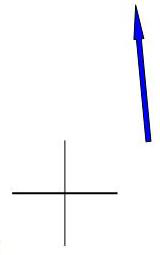In fancy mathematical words, we same that rotation and translation are non-commutative.    A net Affine transformation is thus dependent on not only what operations (rotate, scale, translate) are defined, but also in what order those operations are defined.

## Prototypes

So, what all this boils down to is the following:   In order to put an visual representation of a ball onto the screen, we need to know 3 things:

 Property we need: Where we get it: 1. The scaling of the ball with respect to some "unit" size The ball's radius 2. The rotational orientation of the ball The direction of the ball's velocity 3. Translation from the origin of the display component The ball's x-y location.

With the above 3 things, we can set up an AffineTransform object and it will do all the math to get our ball's visual representation to the correct location on the screen, with the correct size and with the correct orientation!

But what is this "visual representation"?    Notice a key phrase in the above table:  "with respect to some 'unit' size".

All we need to do is to define a unit-sized visual representation of the ball and the affine transform process will take care of sizing it up, orienting it and locating it on the screen.

This unit sized representation is what is called a "prototype" representation of the ball.   We are implementing what is known as the Prototype Design Pattern.  Prototypes are another way in which we can look at the creational process of objects.

Prototypes are objects that are used to as a templates with which to create the actual objects to be used.

So what we will do is to define a unit-sized prototype that the affine transform will use to create a full-sized, properly located and oriented visual representation of the ball.

Luckily, Java provides one interface and one classe that make our lives much easier in this regards (though it would have been even easier if the two were abstractly related!):

java.awt.Shape -- An interface that represents a shape as a set of connected points.  There are many supplied implementations of Shape , including and not limted to, lines, arcs, ellipses, rectangles, polygons, etc.  The points that define these shapes are defined on a double-valued x-y coordinate system.   Thus the unit size (distance) is defined to be 1.0.

java.awt.Image -- A class that represents an image, such as one from a GIF, JPG or PNG file.   Due to the interger coordinate system used to locate pixels in an image, Image objects need to have their unit size defined as the average of the width and height of the image in pixels.   Furthermore, since the useful image may not completely fill the rectangular image area, a "fill factor" needs to be specified that gives the ration of the size of the useful part of the image to the entire image. A fill factor is necessarily always a values less than 1.0.

AffineTransform objects can be used to transform both Shape and Image objects.

### The Painting Process Using Prototypes

First, when the painting strategy is created, the prototype is created ONCE.   The prototype is INVARIANT and thus, is never modified.

Typically we will reuse the `AffineTransform `object rather than reinstantiate it for every use.

We can summarize the painting process using prototypes in these steps, which occur at EVERY painting event:

1. Set the affine transform to translate by the ball's position
2. Add the rotation and scaling to the affine transform as determined by the balls size (radius), velocity and/or other properties at the moment of painting.
3. Add any other desired transformations.
4. Transform the prototype shape into a new `Shape `object which has the desired position, rotation, size, etc.
5. Use the provided `Graphics2D `object to paint the new, transformed `Shape `onto the screen.

### Translating the Prototype Shape ≠ Moving the Ball!

Do not confuse the moving of the ball, which is the changing of the value of the ball's location, with the translation of the prototype shape, which is the generation of the desired shape for painting at the current ball location from the origin-centered prototype shape.

Moving the ball is a completely separate operation from painting the ball.   The ball is painted at the position on the screen corresponding to the current location of the ball.   The ball does not move during painting.    The prototype is invariant and thus never moves from the origin.

The prototype shape is NOT the ball.   The prototype shape contains just the shape information of ball, without any location, size or rotational information.     The location, size and rotational information needed to create the final displayed image of the ball is determined at painting time.   That information is embodied in the affine transform.    Thus, it is the combination of the invariant prototype and the variant affine transform that creates the final shape to be painted on the screen.

© 2019 by Stephen Wong